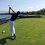# Collaboration set: Number Facts

In collaboration with Kalpok Guha, we made a set of problems based around the topic of "types of numbers". There are many types of numbers out there and here are just a few of them.

This set serves primarially to teach everyone about the complexity of numbers and the many interesting properties they have because math is $\textit{beautiful}$. Except for trig.... Trig is not pretty (lol, I'm bad at trig).

Here is a direct link to the set

here is another one because everyone knows two links are better than 1

Note: most of these problems are doable by hand using a NT approach but a CS approach works just as well.

The "special" numbers include:

Multiplicative perfect numbers- those whose factors when multiplied (including the number itself) yield $n^2$.

Amicable pair- a number$n$ whose factors sum to $k$ and the factors of $k$ sum to $n$.

Abundant number- a number whose factors including itself sum to greater than $2n$.

Deficient number- a number who's factors including itself sum to less than $2n$

Perfect number- a number who's factors including itself sum to $2n$

Narcissistic number- a number of $s$ digits that can be represented by the form $\displaystyle \sum_{i=1}^s 10^{i-1}c_i$ and can be written in the form $\displaystyle \sum_{i=1}^s c_i^n$ where $c$ is a whole number and for some positive integral value of $n$.

Or I'm simplier form, a narcissistic number is one that when each individual digit is summed to the nth power, their sum is the original number.

I must say making this problem set taught me a lot of cool properties about numbers, some that I can't post because I can't think of a problem for them.

I even learned how to spell narcissistic: n-a-r-c-i-s-s-i-s-t-i-c,Note by Trevor Arashiro
6 years, 2 months ago

This discussion board is a place to discuss our Daily Challenges and the math and science related to those challenges. Explanations are more than just a solution — they should explain the steps and thinking strategies that you used to obtain the solution. Comments should further the discussion of math and science.

When posting on Brilliant:

• Use the emojis to react to an explanation, whether you're congratulating a job well done , or just really confused .
• Ask specific questions about the challenge or the steps in somebody's explanation. Well-posed questions can add a lot to the discussion, but posting "I don't understand!" doesn't help anyone.
• Try to contribute something new to the discussion, whether it is an extension, generalization or other idea related to the challenge.
• Stay on topic — we're all here to learn more about math and science, not to hear about your favorite get-rich-quick scheme or current world events.

MarkdownAppears as
*italics* or _italics_ italics
**bold** or __bold__ bold
- bulleted- list
• bulleted
• list
1. numbered2. list
1. numbered
2. list
Note: you must add a full line of space before and after lists for them to show up correctly
paragraph 1paragraph 2

paragraph 1

paragraph 2

[example link](https://brilliant.org)example link
> This is a quote
This is a quote
    # I indented these lines
# 4 spaces, and now they show
# up as a code block.

print "hello world"
# I indented these lines
# 4 spaces, and now they show
# up as a code block.

print "hello world"
MathAppears as
Remember to wrap math in $$ ... $$ or $ ... $ to ensure proper formatting.
2 \times 3 $2 \times 3$
2^{34} $2^{34}$
a_{i-1} $a_{i-1}$
\frac{2}{3} $\frac{2}{3}$
\sqrt{2} $\sqrt{2}$
\sum_{i=1}^3 $\sum_{i=1}^3$
\sin \theta $\sin \theta$
\boxed{123} $\boxed{123}$

## Comments

Sort by:

Top Newest

We have really worked hard on the problems.I have made a set collaboration set So please like and re-share

- 6 years, 2 months ago

Log in to reply

Nice work @Trevor Arashiro and @Kalpok Guha . $\ddot\smile$ I was waiting for so long for this set. Had fun solving all of them.

- 6 years, 2 months ago

Log in to reply

Thank you .

- 6 years, 2 months ago

Log in to reply

@Trevor Arashiro, what you defined in the section of "Narcissistic number" is actually the definition of Perfect Digital Invariant (PDI) and not of Narcissistic number. Note that for a PDI to be a narcissistic number, the power $n$ for the elements of the sum should be equal to the number of digits of the given narcissistic number.

Check out the Wikipedia link on Narcissistic number for details.

- 6 years, 2 months ago

Log in to reply

Yes, you would know what a narcissistic number is :3 lol

But thanks. Learned something once again.

- 6 years, 2 months ago

Log in to reply

I'm not a narcissist in the least, you know. I have almost no self-respect, let alone self admiration. :\ :3

And btw, I think you should edit the note to correct that part.

- 6 years, 2 months ago

Log in to reply

×

Problem Loading...

Note Loading...

Set Loading...